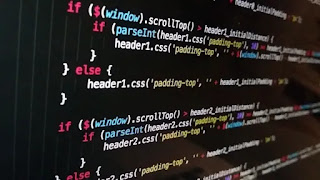# Python else do nothing - codebugfree

In this section we discuss the use of else and why use the else in python programming. Else do nothing or do anything in python program. Else is use as last statement of  if and elif than use the else.

Else is use in program condition. For example if any programmer is make a program with 1 is greater than 2 than use if statement for check the program condition 1 is greater than 2 and print true. If there is 1 is not greater than 2 than print the else statement.

When the programmer only use if than program does not print any thing. If you make he else statement with what programmer want to print that is print in python programming language terminal.python else do nothing

Else is also use in try except. Try except is also known as error handling. Error handling is save your program crash. When the program is run successfully without trace any error than this case print the else statement.

Else statement is optional. Because any case like error handling and condition meet system their is always use else in last. So the codebugfree is opinion that else is do nothing in some case.

Now we see the example of else with optional or not optional. Also see the example of error handling with using the else.

``````first_number = 56
second_number = 10
if first_number==56:
print("here is work the if")
else:
print("here is work else")``````

Her we first make the two variable first number and second number. Making the if else condition checking first number is equal to 56. Yes the first number is equal to 56 and print the if condition.

``here is work the if``

Now we see the else condition .

``````first_number = 56
second_number = 10
if first_number==50:
print("here is work the if")
else:
print("here is work else")
if second_number == 9:
print("printiing the if not printing the else here elelse do nothing")``````

Here is same example as a first. if condition is not meet with if meet the else condition and print else. Again checking the if condition of second number there is no else and not meet with if. So here else do nothing and else is optional.

``````try:
#codebugfree
first_number = int(input("Enter any number: "))
second_number = int(input("Enter any number: "))
multiplying_number = first_number * second_number
print(multiplying_number)
except Exception as e:#treacing the error
e = "Please input a number"#out the error
print(e)
else:
print("you doesnot mistake ")``````

First we make the two variable and get input from user. Multiply the user input. If any case use not input a number than tace the error. If program is run with out error than print else also. Else is optional. If does not use else than your program is not create the big  difference.

INOUTPUT

``````Enter any number: 56
Enter any number: 89
4984
you doesnot mistake``````